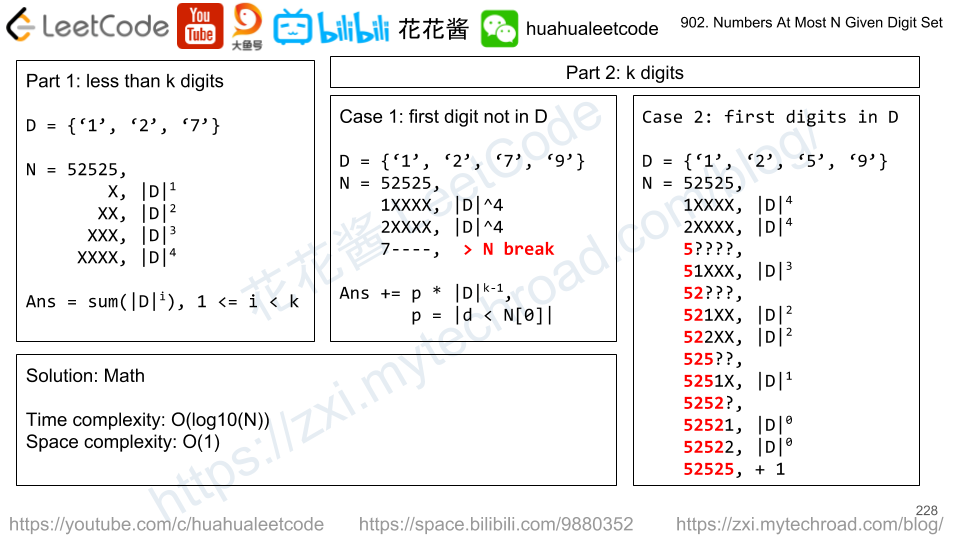# Problem

We have a sorted set of digits D, a non-empty subset of {'1','2','3','4','5','6','7','8','9'}.  (Note that '0' is not included.)

Now, we write numbers using these digits, using each digit as many times as we want.  For example, if D = {'1','3','5'}, we may write numbers such as '13', '551', '1351315'.

Return the number of positive integers that can be written (using the digits of D) that are less than or equal to N.

Example 1:

Input: D = ["1","3","5","7"], N = 100
Output: 20
Explanation:
The 20 numbers that can be written are:
1, 3, 5, 7, 11, 13, 15, 17, 31, 33, 35, 37, 51, 53, 55, 57, 71, 73, 75, 77.


Example 2:

Input: D = ["1","4","9"], N = 1000000000
Output: 29523
Explanation:
We can write 3 one digit numbers, 9 two digit numbers, 27 three digit numbers,
81 four digit numbers, 243 five digit numbers, 729 six digit numbers,
2187 seven digit numbers, 6561 eight digit numbers, and 19683 nine digit numbers.
In total, this is 29523 integers that can be written using the digits of D.

Note:

1. D is a subset of digits '1'-'9' in sorted order.
2. 1 <= N <= 10^9# Solution -1: DFS (TLE)

Time complexity: O(|D|^log10(N))

Space complexity: O(n)

# Solution 1: Math

Time complexity: O(log10(N))

Space complexity: O(1)

Suppose N has n digits.

### less than n digits

We can use all the numbers from D to construct numbers of with length 1,2,…,n-1 which are guaranteed to be less than N.

e.g. n = 52125, D = [1, 2, 5]

format X: e.g. 1, 2, 5 counts = |D| ^ 1

format XX: e.g. 11,12,15,21,22,25,51,52,55, counts = |D|^2

format XXX:  counts = |D|^3

format XXXX: counts = |D|^4

### exact n digits

if all numbers in D  != N, counts = |d < N | d in D| * |D|^(n-1), and we are done.

e.g. N = 34567, D = [1,2,8]

we can make:

• X |3|^1
• XX |3| ^ 2
• XXX |3| ^ 3
• XXXX |3| ^ 3
• 1XXXX, |3|^4
• 2XXXX, |3|^4
• we can’t do 8XXXX

Total = (3^1 + 3^2 + 3^3 + 3^4) + 2 * |3|^ 4 = 120 + 162 = 282

N = 52525, D = [1,2,5]

However, if d = N[i], we need to check the next digit…

• X |3|^1
• XX |3| ^ 2
• XXX |3| ^ 3
• XXXX |3| ^ 3
• 1XXXX, |3|^4
• 2XXXX, |3|^4
•  5????
• 51XXX |3|^3
• 52???
• 521XX |3|^2
• 522XX |3|^2
• 525??
• 5251X |3|^1
• 5252?
• 52521 |3|^0
• 52522 |3|^0
• 52525 +1

total = (120) + 2 * |3|^4 + |3|^3 + 2*|3|^2 + |3|^1 + 2 * |3|^0 + 1 = 120 + 213 = 333

if every digit of N is from D, then we also have a valid solution, thus need to + 1.

## Python3

If you like my articles / videos, donations are welcome.

Buy anything from Amazon to support our website## Be First to Comment

Mission News Theme by Compete Themes.